﻿ 单/双层水下结构振动声辐射研究
 舰船科学技术2017, Vol. 39Issue (1): 17-20PDF

1. 海军工程大学 科研部，湖北 武汉 430033;
2. 船舶振动噪声重点实验室，湖北 武汉 430033;
3. 海军工程大学 动力工程学院，湖北 武汉 430033

Research on the vibration and acoustic radiation of single and double layers underwater structure
HE Qi-wei1,2, LI Hai-feng3, YU Xiang1,2
1. Office of Research and Design, Naval University of Engineering, Wuhan 430033, China;
2. National Key Laboratory on Ship Vibration and Noise, Wuhan 430033, China;
3. College of Power Engineering, Naval University of Engineering, Wuhan 430033, China
Abstract: To research the vibration and acoustic radiation characteristics of the single-layer and double-layer underwater structure which are the typical structures of the submarine, the single-layer and double-layer propeller-shaft-hull coupled structures are established, the three-dimensional sono-elastical theory and acoustic analysis software are applied to analyze the sound source level curve of the coupled structure under three different exciting such as propeller exciting, external fluid exciting and internal mechanical exciting. The analysis results show that the total radiated acoustic power of single-layer underwater structure is less than double-layer under propeller exciting, but higher than double-layer under external fluid exciting and internal mechanical exciting.
Key words: Single and double layers structure     propeller-shaft-hull     vibration     acoustic radiation     sound source level
0 引言

1 三维声弹性力学理论

 ${u} = \sum\limits_{r = 1}^m {{{u}_r}{q_r}} = \sum\limits_{r = 1}^m {\left\{ {{u_r},{v_r},{w_r}} \right\}{q_r}},$ (1)

 $\Phi \left( {x,y,z,t} \right) = \sum\limits_{r = 1}^m {{\phi _r}\left( {x,y,z,t} \right)} ,$ (2)

 ${\nabla ^2}\phi- \frac{{{\partial ^2}\phi }}{{\partial {t^2}}} = 0,$ (3)

 $\frac{{\partial {\phi _r}}}{{\partial {{n}}}} = iw\left( {{u_r}{n_1} + {v_r}{n_2} + {w_r}{n_3}} \right)。$ (4)

 $G\left( {P,Q} \right) = \frac{1}{{4\pi {r_1}}}{e^{- ik{r_1}}}- \frac{1}{{4\pi {r_2}}}{e^{- ik{r_2}}}。$ (5)

 $\phi \left( P \right) = \frac{1}{{4\pi }}\int \!\!{\int_{\bar S} {\sigma \left( Q \right)G\left( {P,Q} \right){\rm{d}}{S_Q}} } ,$ (6)

 $\left[ {a + A} \right]\left\{ {\ddot q} \right\} + \left[ {b + B} \right]\left\{ {\dot q} \right\} + \left[ {c + C} \right]\left\{ q \right\} = \left\{ \Xi \right\}$ (7)

 $\left. {\begin{array}{*{20}{l}} {{A_{rk}}}\\ {{B_{rk}}} \end{array}} \right\} = \left. {\begin{array}{*{20}{l}} {\frac{1}{{{\omega ^2}}}{\rm{Re}}}\\ {- \frac{1}{\omega }{\rm{Im}}} \end{array}} \right\}\left[ {\rho \int \!\!{\int_{\bar S} {{ n} \cdot { u_r}\left( {i\omega } \right){\phi _k}{\rm{d}}S} } } \right],$ (8)
 ${C_{rk}} =- \rho \int_{\bar S} { n \cdot { u_r} \cdot g \cdot {\omega _k}{\rm{d}}S} ,$ (9)

 $\begin{array}{l} p\left( {x,y,z,t} \right) =- \rho \sum\limits_{r = 1}^m {\frac{{\partial {\phi _r}}}{{\partial t}}} =- \rho \sum\limits_{r = 1}^m {\left( {i\omega } \right)} \times \\ \;\;\;\;\;\;\;\;\;\;\;\;\;\;\;\;\;\;\;\;\;\;\left( {\frac{1}{{4\pi }}{{\left( {\int {\int_{\bar S} {\sigma \left( Q \right)G\left( {P,Q} \right)dS} } } \right)}_r}} \right)。\end{array}$ (10)
2 桨-轴-壳体计算模型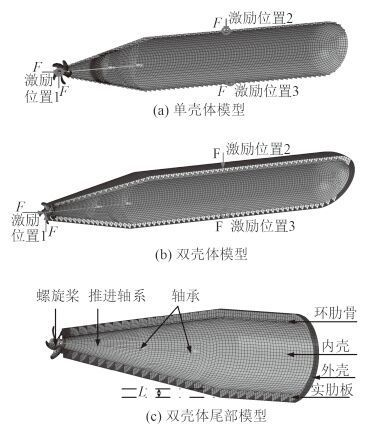图 1 计算模型 Fig. 1 Calculation model
3 数值计算及结果分析

 ${L_s} = 10\lg \frac{{P/\left( {4\pi } \right)}}{{{P_0}}}。$ (11)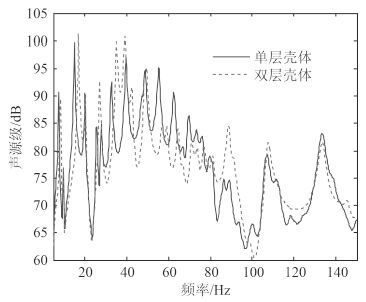图 2 螺旋桨横向激励下单/双层结构声源级曲线 Fig. 2 Sound source level curve of single and double layers structures under propeller vertical exciting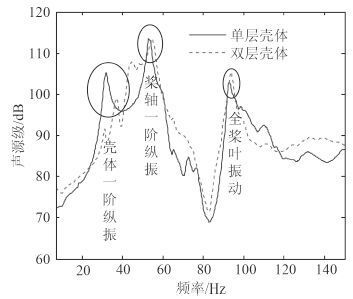图 3 螺旋桨纵向激励下单/双层结构声源级曲线 Fig. 3 Sound source level curve of single and double layers structures under propeller longitudinal exciting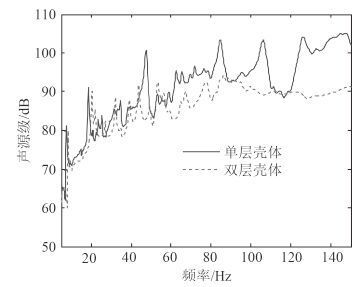图 4 外部水噪声激励下单/双层结构声源级曲线 Fig. 4 Sound source level curve of single and double layers structures under external fluid exciting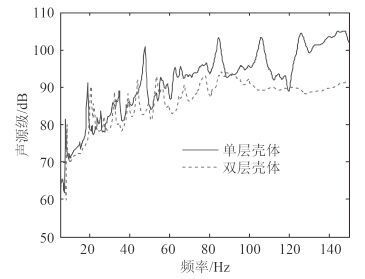图 5 内部机械激励下单/双层结构声源级曲线 Fig. 5 Sound source level curve of single and double layers structures under internal mechanical exciting
4 结语

1）在螺旋桨横向力和纵向力作用下，单/双层水下结构声源级曲线相互交叉，声辐射相差不大，在 10～150 Hz 频段内单层水下结构声辐射总声级略低于双层结构。

2）在外部流体激励力和内部机械设备激励力作用下，单层结构声辐射声源级曲线高于双层结构，因此，在 10～150 Hz 频段内单层水下结构声辐射总声级明显高于双层结构。

  祁立波, 邹明松. 加肋圆柱体水下低频辐射声特性研究[J]. 船舶力学 , 2015, 19 (7):874–883. Qi Li-bo, ZOU Ming-song. Research on acoustic radiation of stiffened cylinder in low-frequency[J]. Journal of Ship Mechanics , 2015, 19 (7) :874–883.  夏齐强, 陈志坚. 降低加肋双层圆柱壳辐射噪声线谱的结构声学设计[J]. 声学学报 , 2014, 39 (5):613–623. XIA Ji-qiang, CHEN Zhi-jian. Structural-Acoustic design to depress line spectrum of radiation noise from double cylindrical ring stiffened shell[J]. Acta Acustica , 2014, 39 (5) :613–623.  金广文, 姜荣俊, 陈美霞, 等. 不同参数下的单、双层圆柱壳体速度场研究[J]. 振动与冲击 , 2006, 25 (3):169–175. JIN Guang-wen, JIANG Rong-jun, CHEN Mei-xia. Velocity field of finite cylindrical shells with single or double layers and different parameters[J]. Journal of Vibration and Shock , 2006, 25 (3) :169–175.  唐俊娟, 刘艳敏. 单双层壳舱段结构声辐射计算分析[C]//. 第十四届船舶水下噪声学术讨论会论文集.  李兵, 张超. 单/双层圆柱壳振动及声辐射对比[J]. 舰船科学技术 , 2015, 37 (2):14–18. LI Bing, ZHANG Chao. Comparision on vibration and sound radiation from single and double cylindrical shells[J]. Ship Science and Technology , 2015, 37 (2) :14–18.  WU Y S. Hydroelasticity of floating bodies[D]. London: Brunel University, 1984.  ZOU M S, WU Y S, YE Y L. Three-dimensional hydroelasticity analysis of acoustic responses of ship structures[C]//. 9th International Conference on Hydrodynamics, 2010, 10: 844-851.